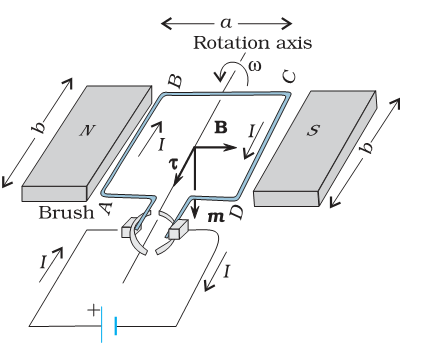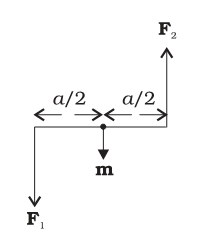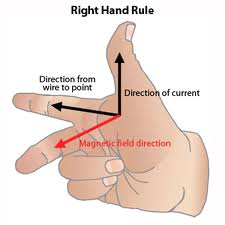1800-212-7858 (Toll Free)
9:00am - 8:00pm IST all days
8104911739

or

Thanks, You will receive a call shortly.
Customer Support

You are very important to us

022-62211530

Mon to Sat - 11 AM to 8 PM

# question

Asked by 30th July 2012, 6:39 AM
Electric Motor:

It works on the principle of force applied on a current carrying conductor due a magnetic field.
When a curent carrying conductor is kept in an electric field it experiences a force in the direction of cross product of the force and field, that force can be shown as: F = iLxB
here L is the vector showing direction of current and B is the magnetic field.
In many cases it can be simplfied using the left hand rule:
Stretch the middle finger, forefinger and the thumb of your left hand mutually perpendicular to each other then if forefinger represents the direction of magnetic field, middle finger represents the direction of current then the thumb will represent the direction of force on the conductor.Now we can apply the above principle to the given system called electric motor. It is used to convert electrical energy to rotational energy. and consists of a coil on an axle inside a magnetic field:When we apply the above rule to each side of coil, we get the forces in following direction:Thus this couple of force rotates the coil about the axis.
But after one rotation it creates a problem. If the current is allowed to flow in the same direction after half rotation, the direction of forces reverses. Thus cant be allowed to make a complete rotation, this we need to reverse the direction of current and we do it by using a commutator and carbon brushes.
It consist of following assembly:Thus after each half rotation, the direction of current is reversed.

Electric generator on the other hand works on an exactly opposite principle.
When a conductor is moved in a magnetic field, it generates an emf across the conductor and if it is joined to a resistance, a current flows in the conductor.
It can be shown by the right hand rule:Thus if the above shown figure of electric motor is rotated, an emf is generated across the two input leads by  the same principle.
Answered by Expert 4th August 2012, 12:20 PM
• 1
• 2
• 3
• 4
• 5
• 6
• 7
• 8
• 9
• 10

You have rated this answer /10

RELATED STUDY RESOURCES :